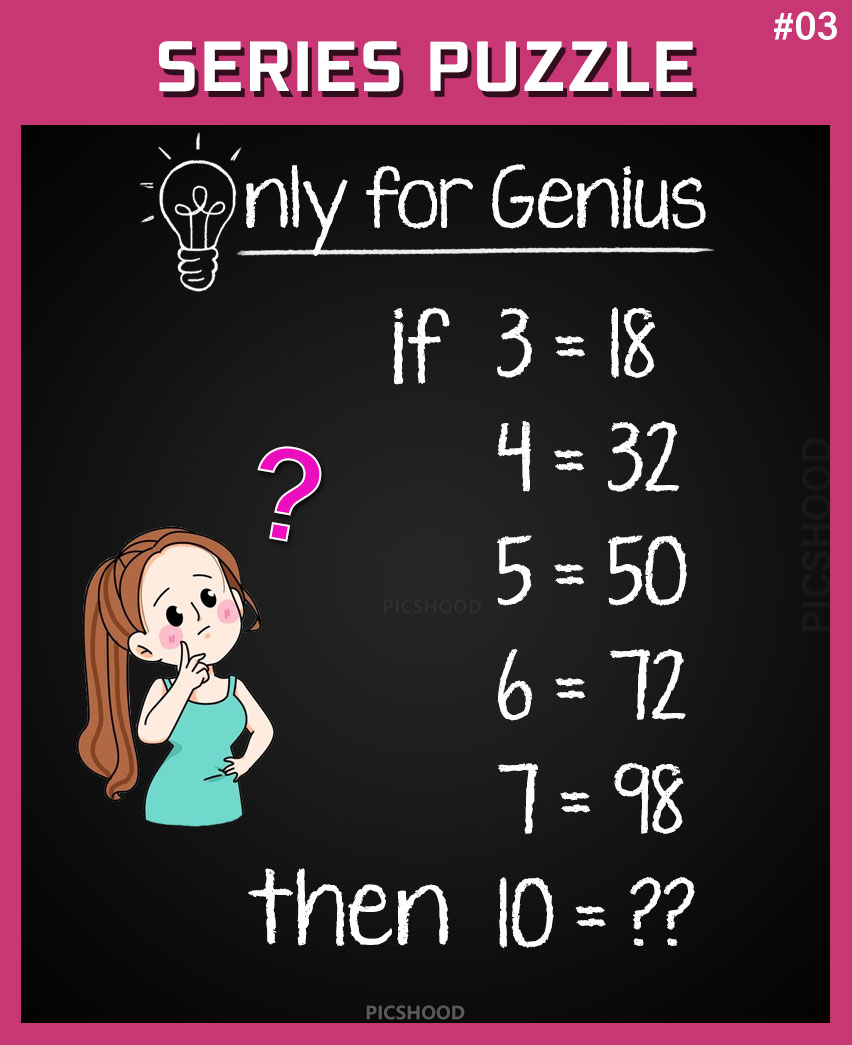This post may contain affiliate links. This means if you click on the link and purchase the item, We will receive an affiliate commission at no extra cost to you. See Our Affiliate Policy for more info.

if 3=18, 4=32, 5=50, then 10=?? Only for Genius – Number Series Puzzle #3

2
6220

Hello and welcome back to the number series puzzle. Another interesting “Only for Genius Math Puzzle” getting viral on social media.

In these kind of puzzles you have to find out the logic behind this puzzle and then you will get the correct answer. Let see if you can find the pattern of this math problem.

Logical Number Series Puzzle Question:

• If 3=18
• 4=32
• 5=50
• 6=72
• 7=98
• Then 10=??Did you found the pattern of the puzzle? Share you answer in the comments before confirm.

Solution:-

Here is a Pattern to solve this math puzzle;

• 3 = 18   ( 3 * 6 = 18 )
• 4 = 32   ( 4 * 8 = 32 )
• 5 = 50   ( 5 * 10 = 50 )
• 6 = 72   ( 6 * 12 = 72 )
• 7 = 98   ( 7 * 14 = 98 )

8 and 9 are Missing in the puzzle.

• 8 = 128  ( 8 * 16 = 128 )
• 9 = 162  ( 9 * 18 = 162 )

So, Then  10 = 200  ( 10 * 20 = 200 )

If You See another Pattern or Solution Please Tell Us in Comment Below!

Hope you found this genius puzzle interesting, don’t forget to share it. Connect with us for more viral and amazing brainteasers.

Enjoy and Take care!

1.jackie marshall
2.Uzma